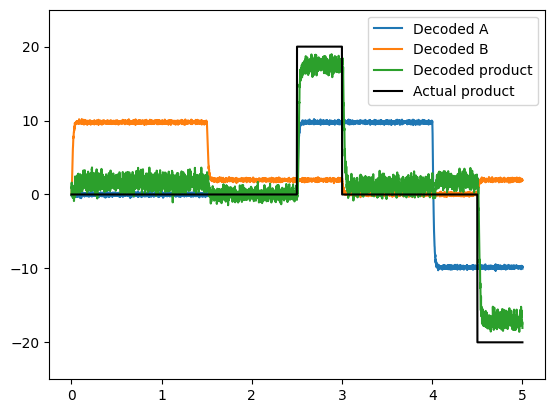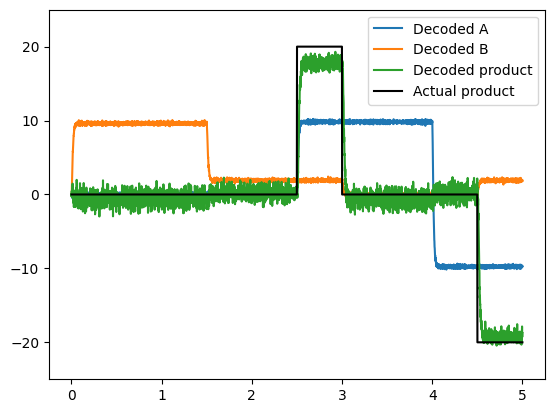Note

This documentation is for a development version. Click here for the latest stable release (v4.0.0).

# Multiplication¶

This example will show you how to multiply two values. The model architecture can be thought of as a combination of the combining demo and the squaring demo. Essentially, we project both inputs independently into a 2D space, and then decode a nonlinear transformation of that space (the product of the first and second vector elements).

:

%matplotlib inline
import matplotlib.pyplot as plt
import numpy as np

import nengo
from nengo.dists import Choice
from nengo.processes import Piecewise


## Step 1: Create the model¶

The model has four ensembles: two input ensembles (‘A’ and ‘B’), a 2D combined ensemble (‘Combined’), and an output ensemble (‘D’).

:

# Create the model object
model = nengo.Network(label="Multiplication")
with model:
# Create 4 ensembles of leaky integrate-and-fire neurons
combined = nengo.Ensemble(
)  # This radius is ~sqrt(10^2+10^2)

# This next two lines make all of the encoders in the Combined population
# point at the corners of the cube.
# This improves the quality of the computation.

# Comment out the line below for 'normal' encoders
combined.encoders = Choice([[1, 1], [-1, 1], [1, -1], [-1, -1]])


## Step 2: Provide input to the model¶

We will use two varying scalar values for the two input signals that drive activity in ensembles A and B.

:

with model:
# Create a piecewise step function for input
inputA = nengo.Node(Piecewise({0: 0, 2.5: 10, 4: -10}))
inputB = nengo.Node(Piecewise({0: 10, 1.5: 2, 3: 0, 4.5: 2}))

correct_ans = Piecewise({0: 0, 1.5: 0, 2.5: 20, 3: 0, 4: 0, 4.5: -20})


## Step 3: Connect the elements of the model¶

:

with model:
# Connect the input nodes to the appropriate ensembles
nengo.Connection(inputA, A)
nengo.Connection(inputB, B)

# Connect input ensembles A and B to the 2D combined ensemble
nengo.Connection(A, combined)
nengo.Connection(B, combined)

# Define a function that computes the multiplication of two inputs
def product(x):
return x * x

# Connect the combined ensemble to the output ensemble D
nengo.Connection(combined, prod, function=product)


## Step 4: Probe the output¶

Collect output data from each ensemble and input.

:

with model:
inputA_probe = nengo.Probe(inputA)
inputB_probe = nengo.Probe(inputB)
A_probe = nengo.Probe(A, synapse=0.01)
B_probe = nengo.Probe(B, synapse=0.01)
combined_probe = nengo.Probe(combined, synapse=0.01)
prod_probe = nengo.Probe(prod, synapse=0.01)


## Step 5: Run the model¶

:

# Create the simulator
with nengo.Simulator(model) as sim:
# Run it for 5 seconds
sim.run(5)


## Step 6: Plot the results¶

To check the performance of the model, we can plot the input signals and decoded ensemble values.

:

# Plot the input signals and decoded ensemble values
plt.figure()
plt.plot(sim.trange(), sim.data[A_probe], label="Decoded A")
plt.plot(sim.trange(), sim.data[B_probe], label="Decoded B")
plt.plot(sim.trange(), sim.data[prod_probe], label="Decoded product")
plt.plot(
sim.trange(), correct_ans.run(sim.time, dt=sim.dt), c="k", label="Actual product"
)
plt.legend(loc="best")
plt.ylim(-25, 25)

:

(-25.0, 25.0)The input signals we chose make it obvious when things are working, as the inputs are zero often (so the product should be). When choosing encoders randomly around the circle (the default in Nengo), you may see more unwanted interactions between the inputs. To see this, comment the above code that sets the encoders to the corners of the cube (in Step 1 where it says # Comment out the line below for 'normal' encoders).

## Bonus step: Make a subnetwork¶

If you find that you need to compute the product in several parts of your network, you can put all of the components necessary to compute the product together in a subnetwork. By making a function to construct this subnetwork, it becomes easy to make many such networks in a single model.

:

def Product(neuron_per_dimension, input_magnitude):
# Create the model object
model = nengo.Network(label="Product")
with model:
# Create passthrough nodes to redirect both inputs
model.A = nengo.Node(output=None, size_in=1)
model.B = nengo.Node(output=None, size_in=1)

model.combined = nengo.Ensemble(
neuron_per_dimension * 2,
dimensions=2,
encoders=Choice([[1, 1], [-1, 1], [1, -1], [-1, -1]]),
)

model.prod = nengo.Ensemble(
)

# Connect everything up
nengo.Connection(model.A, model.combined, synapse=None)
nengo.Connection(model.B, model.combined, synapse=None)

def product(x):
return x * x

nengo.Connection(model.combined, model.prod, function=product)
return model

# The previous model can then be replicated with the following
model = nengo.Network(label="Multiplication")
with model:
inputA = nengo.Node(Piecewise({0: 0, 2.5: 10, 4: -10}))
inputB = nengo.Node(Piecewise({0: 10, 1.5: 2, 3: 0, 4.5: 2}))
prod = Product(100, input_magnitude=10)
nengo.Connection(inputA, A)
nengo.Connection(inputB, B)
nengo.Connection(A, prod.A)
nengo.Connection(B, prod.B)

inputA_probe = nengo.Probe(inputA)
inputB_probe = nengo.Probe(inputB)
A_probe = nengo.Probe(A, synapse=0.01)
B_probe = nengo.Probe(B, synapse=0.01)
combined_probe = nengo.Probe(prod.combined, synapse=0.01)
prod_probe = nengo.Probe(prod.prod, synapse=0.01)

# Create the simulator
with nengo.Simulator(model) as sim:
# Run it for 5 seconds
sim.run(5)

# Plot the input signals and decoded ensemble values
plt.figure()
plt.plot(sim.trange(), sim.data[A_probe], label="Decoded A")
plt.plot(sim.trange(), sim.data[B_probe], label="Decoded B")
plt.plot(sim.trange(), sim.data[prod_probe], label="Decoded product")
plt.plot(
sim.trange(), correct_ans.run(sim.time, dt=sim.dt), c="k", label="Actual product"
)
plt.legend(loc="best")
plt.ylim(-25, 25)

:

(-25.0, 25.0)Alternatively, you can use Nengo’s built in nengo.networks.Product network <https://www.nengo.ai/nengo/networks.html#nengo.networks.Product>__. This network works with input of any dimensionality (e.g., to compute the dot product of two large vectors) and uses special optimizations to make the product more accurate than this implementation.